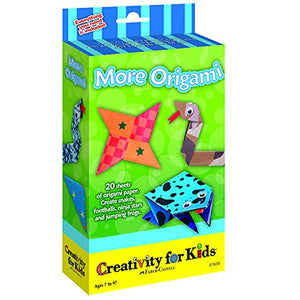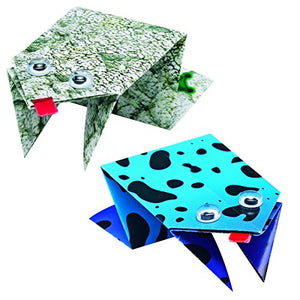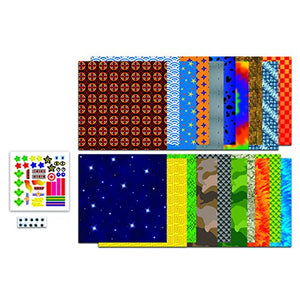# Creativity For Kids More Origami

Vendor
Creativity for Kids
Regular price
\$31.06
Sale price
\$31.06
Quantity must be 1 or more

• F

• a

• b

• e

• r

• -

• C

• a

• s

• t

• e

• l

• l

• C

• r

• e

• a

• t

• i

• v

• i

• t

• y

• f

• o

• r

• K

• i

• d

• s

• M

• o

• r

• e

• O

• r

• i

• g

• a

• m

• i

• A

• r

• t

• s

• &

• C

• r

• a

• f

• t

• s

Faber-Castell Creativity for Kids More Origami